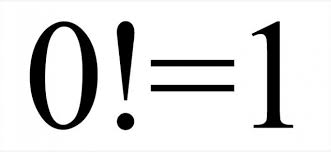## Wednesday, 20 May 2015

### Why 0! = 1

Why 0 Factorial (0!) is equal to 15! = 5*4*3*2*1 = 120
4! = 4*3*2*1 = 24

4! is also = 5! / 5, because 4! has all in 5! but the five, so simply divide by five. Now let's continue a bit more..

3! = 4!/4 = 6
3! = 3*2*1 = 6
2! = 3!/3 = 2
2! = 2*1 = 2
1! = 2!/2 = 1
1! = 1 = 1

Now if you look at 0!, this is the reason it is equal to 1..

0! = 1!/1 which is 1/1, which is 1
therefore 0! is 1

You can also go as far as -1! if you'd like
-1! = 0!/0 which is 1/0
-1! = undefined, infinity, etc..